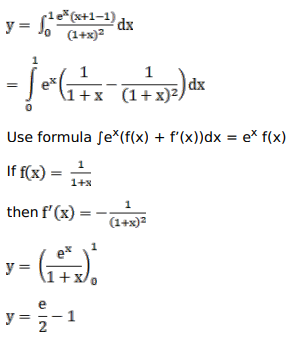# Mark against the correct answer in the following:

Question:

Mark $(\sqrt{)}$ against the correct answer in the following:

$\int_{0}^{1} \frac{x e^{x}}{(1+x)^{2}} d x=?$

A. $\left(\frac{\mathrm{e}}{2}-1\right)$

B. $(e-1)$

C. $e(e-1)$

D. none of these

Solution: